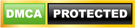# Watch Calculator and Bulb Puzzle: check out the Watch Calculator Bulb Puzzle answer or Clock Calculator Bulb Puzzle Answer

Watch Calculator and Bulb Puzzle: Watch Calculator Bulb Puzzle is one of the puzzles that will really test your Math Skills. If you really think you are good at Math, try this Clock Calculator and Bulb Puzzle and test yourself. Share the Watch Calculator Bulb Puzzle with your friend and family and challenge them. Check out the solution for the Watch Calculator Bulb Puzzle answer in this article.

Updated: Jun 09, 2020 17:43 ISTSuganya Vedham

## What is Watch Calculator and Bulb Puzzle?

Amid the COVID-19 lockdown, people have nothing to do during their excess free time. The spare you from the boredom, you can try online puzzles, games, and quizzes to sharpen and challenge your skills. Some people have started solving riddles and puzzles on social media to stay active. There are many mind-tricking riddles and puzzles that will really sharpen your wits during the pandemic. One such puzzle that challenged people and has gone viral on social media is the 'watch calculator bulb' puzzle. It is currently trending on Whatsapp and social media.

## Watch Calculator Bulb Puzzle:

Check out the puzzle that will improve your creative thinking and math skills.You should know that the puzzle is tricky and difficult. The puzzle not only tests your math skills but also makes you think creatively by correlating household electronics with math equations.

Here is a tip for you! You need to pay attention to the minute details of each electronic device, such as the time on the clock, the numbers on the calculator, and the light rays coming out of the bulb. Only by considering all these factors, you can solve the Watch Calculator bulb puzzle.

## What is the answer for Watch Calculator and Bulb Puzzle?

Well, solving the Watch Calculator Bulb Puzzle wasn't easy as you thought, was it? If you have found the answer verify it with the correct answer given below. Here is your right answer.

Answer to the Watch Calculator bulb puzzle is 333.

Take the first clock equation. you have to find the value of one clock using the first equation.
According to first equation,
9'o clock + 9'o clock + 3'o clock = 9 + 9 + 3 = 21.
Therefore, 1 Clock = 1

Now consider the second equation. In the second equation, three calculators are equal to 30. Therefore, one calculator is equal to 10. The value of the calculator depends on the sum of the numbers inside it.
Hence, the sum of numbers mention inside the calculator "1234" is:
1 + 2 + 3 + 4 = 10.

In the third equation shows that 1 Bulb + 1 Bulb – 1 Bulb = 15.
Therefore, one bulb with one light ray = 3
Bulb with 5 light rays = 5 x 3 = 15
THerefore, 15 + 15 - 15 = 15

The final equation, to solve, is 9’o clock + Calulator (1+2+2+4) x 3 bulbs (with four light rays each)
Converting the equation into numbers, we get,
9 + (1+2+2+4) x 3(4x3)
9 + 9 x 3(12)
9 + 9 x 36 = 9 + 324 = 333
Answer to 'Watch Calculator bulb' puzzle is 333.

## Watch Calculator and Bulb Puzzle - FAQ

1. What are the uses of Maths challenges?

Maths games and activities can help you stay active and enthusiastic for numeracy. Such a puzzle takes away the fear of numbers. It helps to grow diverse mathematical concepts and develop strategic thinking. Above all, it improves learning skills.

2. What are skills can puzzle-solving develop?

Solving puzzles helps ro develop basic skills like shape recognition, goal setting, concentration, patience, problem-solving and decision making skills, and a sense of achievement. Puzzles also help to develop hand-eye coordination and fine motor skills due to the precise nature of matching each piece exactly.